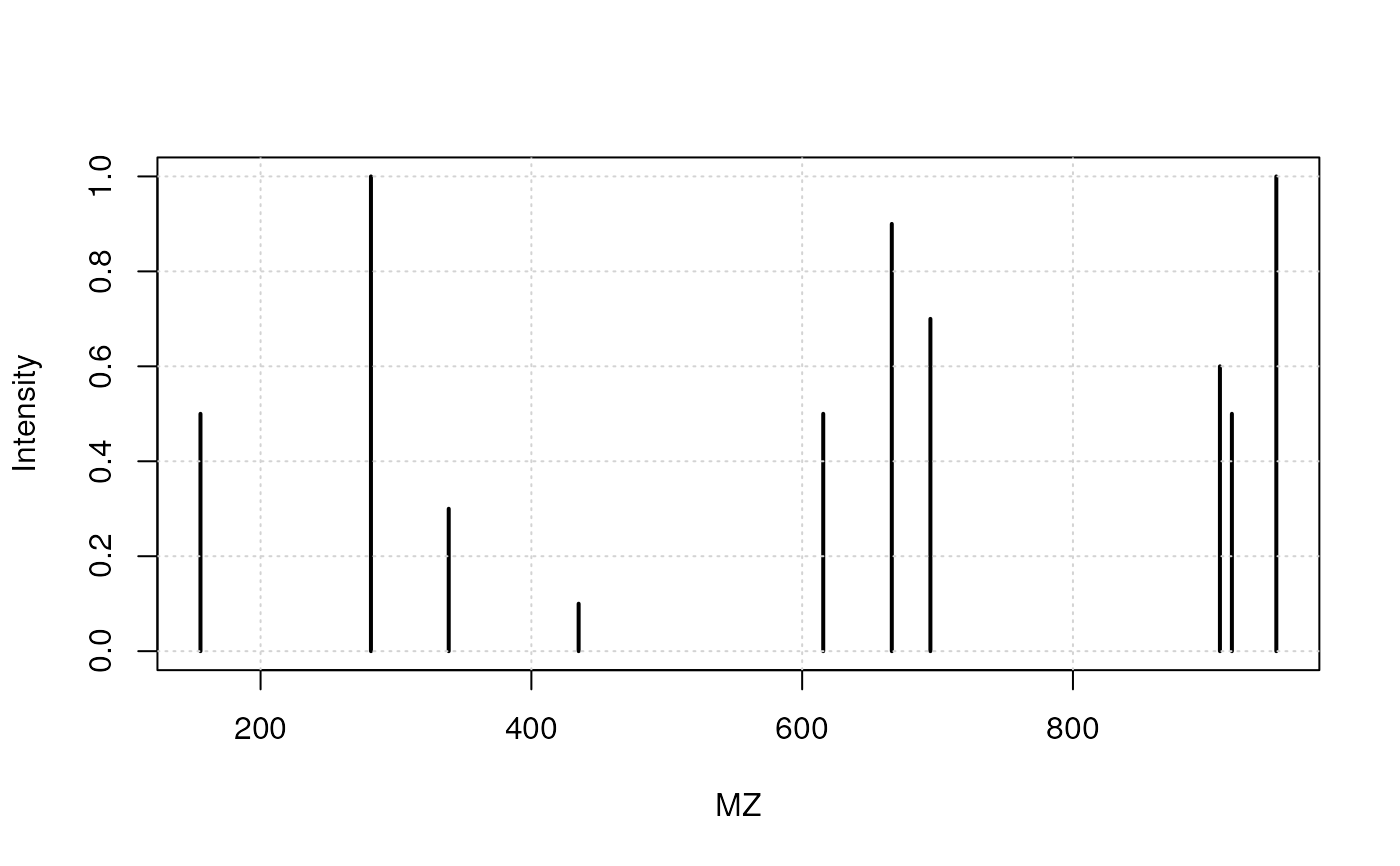This is the constructor function to generate a set of ions that can later be analysed with analyse() and detected with detect().

new_ions(npeaks = 10, mzrange = c(100, 1000), nimg = 100)

analyse(x, sleep = 0.1)

analyze(x, sleep = 0.1)

detect(x, new = FALSE)

spectrum(x, ...)

## Arguments

npeaks

A numeric scalar defining the number of unique peaks (M/Z values). Default is 10.

mzrange

A numeric of length 2 defining the range of possible M/Z values. Default is c(100, 1000).

nimg

A numeric scalar. When analysing the ions, their separation along their M/Z values will be split along a sequence of length nimg. Default is 100.

x

An object of class ions.

sleep

How much time to wait before producing the next plot.

new

A logical scalar, indicating if the separated ions (last frame of calling analyse) should be plotting, or whether the detection should be overlaid. Default is FALSE, to add the plot on top of the opened device.

...

## Value

An object of class ions.

analyse, detect and spectrum are used for their side effect or producing plots. They all invisibly return NULL.

Laurent Gatto

## Examples

set.seed(1L)
x <- new_ions(nimg = 5)
x
#> Object of class 'ions':
#> # analyze(.); detect(.); spectrum(.)
analyse(x)detect(x)spectrum(x)`